## How to Calculate and Solve for Mass, Height and Potential Energy | The Calculator Encyclopedia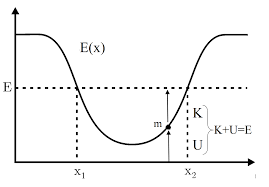The image above represents potential energy.

To compute for the potential energy, three essential parameters are needed and these parameters are mass (m), height (h) and acceleration due to gravity (g).

The formula for calculating the potential energy:

P.E = mgh

Where;
P.E. = Potential Energy
m = Mass
g = acceleration due to gravity
h = Height

Let’s solve an example;
Find the potential energy when the mass is 12 with a height of 24 and acceleration due to gravity of 9.8.

This implies that;
m = Mass = 12
g = acceleration due to gravity = 9.8
h = Height = 24

P.E = mgh
P.E = 12 x 9.8 x 24
P.E = 2822.4

Therefore, the potential energy is 2822.4 Joules (J).

Calculating the Mass when Potential Energy, Height and Acceleration due to Gravity is Given.

m = P.E / gh

Where;
m = Mass
P.E. = Potential Energy
g = acceleration due to gravity
h = Height

Let’s solve an example;
Find the Mass when potential energy is 450 with a height of 30 and acceleration due to gravity of 10.

This implies that;
P.E. = Potential Energy = 450
g = acceleration due to gravity = 10
h = Height = 30

m = P.E / gh
m = 450 / 10 x 30
m = 450 / 300
m = 1.5

Therefore, the mass is 1.5.

## How to Calculate and Solve for Mass, Velocity and Kinetic Energy | The Calculator Encyclopedia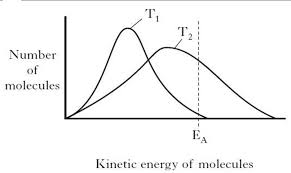The image above represents kinetic energy.

To compute for the kinetic energy, two essential parameters are needed and these parameters are mass (m) and velocity (v).

The formula for calculating the kinetic energy:

K.E. = 0.5mv²

Where;
K.E. = Kinetic Energy
m = Mass
v = Velocity

Let’s solve an example;
Find the kinetic energy when the mass is 6 and the velocity is 18.

This implies that;
m = Mass = 6
v = Velocity = 18

K.E. = 0.5mv²
K.E. = 0.5[6 x 18²]
K.E. = 0.5[6 x 324]
K.E. = 0.5
K.E. = 972

Therefore, the kinetic energy is 972 Joules (J).

Calculating the Mass when Kinetic Energy and Velocity is Given.

m = K.E / 0.5v2

Where;
m = Mass
K.E. = Kinetic Energy
v = Velocity

Let’s solve an example;
Find the mass when the kinetic energy is 320 and a velocity of 20.

This implies that;
K.E. = Kinetic Energy = 320
v = Velocity = 20

m = K.E / 0.5v2
m = 320 / 0.5 x 202
m = 320 / 0.5 x 40
m = 320 / 20
m = 16

Therefore, the mass is 16 kg.

## How to Calculate and Solve for Mass, Volume and Linear Momentum | The Calculator Encyclopedia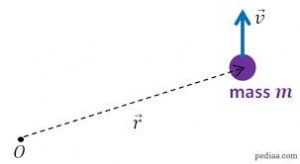The image above represents a linear momentum.

To compute for the linear momentum, two essential parameters are needed and these parameters are mass (m) and velocity (v).

The formula for calculating linear momentum:

p = mv

Where;
p = Momentum
m = Mass
v = Velocity

Let’s solve an example;
Find the linear momentum of a mass of 44 and a velocity of 38.

This implies that;
m = Mass = 44
v = Velocity = 38

p = mv
p = 44 x 38
p = 1672

Therefore, the linear momentum is 1672 Kgm/s.

Calculating the Mass when Linear Momentum and Velocity is Given.

m = p / v

Where;
m = Mass
p = Momentum
v = Velocity

Let’s solve an example;
Find the mass with a linear momentum of 320 and a velocity of 80.

This implies that;
p = Momentum = 320
v = Velocity = 80

m = p / v
m = 320 / 80
m = 4

Therefore, the mass is 4 kg.

## How to Calculate and Solve for the Quantity of Charge, Electrochemical Equivalence of a Substance and Mass of an Element in Electrolysis | Nickzom Calculator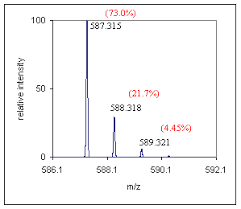The image above represents the mass of an element.

To compute for the mass of an element, two essential parameters are needed and these parameters are Electrochemical Equivalence of the Substance (Z) and quantity of charge (Q).

The formula for calculating mass of an element:

M = ZQ

Where;
M = Mass of the element
Z = Electrochemical Equivalence of the Substance
Q = Quantity of Charge

Let’s solve an example;
Find the mass of an element when the Quantity of charge is 28 and Electrochemical Equivalence of the Substance is 32.

This implies that;
Z = Electrochemical Equivalence of the Substance = 32
Q = Quantity of Charge = 28

M = ZQ
M = 32 x 28
M = 896

Therefore, the mass of an element is 896 kg.

Calculating the Electrochemical Equivalence of the Substance when the Mass of an Element and Quantity of Charge is Given.

Z = M / Q

Where;
Z = Electrochemical Equivalence of the Substance
M = Mass of the element
Q = Quantity of Charge

Let’s solve an example;
Find the Electrochemical Equivalence of the Substance when the Quantity of charge is 12 and  mass of an element  is 120.

This implies that;
M = Mass of the element = 120
Q = Quantity of Charge = 12

Z = M / Q
Z = 120 / 12
Z = 10

Therefore, the Electrochemical Equivalence of the Substance is 10.

## How to Calculate and Solve for the Current, Time and Quantity of Charge of an Electrolysis | The Calculator Encyclopedia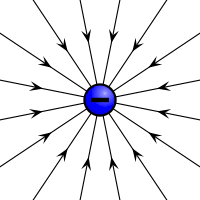The image above represents the quantity of charge.

To compute for the quantity of charge, two essential parameters are needed and these parameters are current (I) and time (T).

The formula for calculating the quantity of charge:

Q = It

Where;
Q = Quantity of charge
I = Current
T = Time

Let’s solve an example;
Find the quantity of charge with a current of 24 and time of 12.

This implies that;
I = Current = 24
T = Time = 12

Q = It
Q = 24 x 12
Q = 288

Therefore, the quantity of charge is 288 Coulombs (C).

Calculating the Current (I) using the Quantity of Charge and Time.

I = Q / t

Where;
I = Current
Q = Quantity of charge
T = Time

Let’s solve an example;
Given that the quantity of charge is 240 with a time of 14. Find the Current?

This implies that;
Q = Quantity of charge = 240
T = Time = 14

I = Q / t
I = 240 / 14
I = 17.14

Therefore, the current is 17.14 ampere.

## How to Calculate and Solve for Van’t Hoff Factor, Ebullioscopic Constant, Molality and Boiling Point Elevation | The Calculator Encyclopedia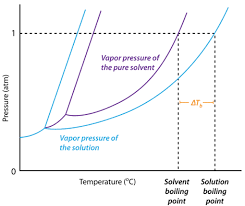The image above represents boiling point elevation.

To compute for the boiling point elevation, three parameters are needed and these parameters are Van’t Hoff’s Factor (i), ebullioscopic constant (Kb) and Molality.

The formula for calculating boiling point elevation:

δTb = iKb x Molality

Where;
δTb = boiling point elevation
i = Van’t Hoff’s Factor
Kb = ebullioscopic constant
Molality

Let’s solve an example;
Find the boiling point elevation when the Van’t Hoff’s Factor is 42, ebullioscopic constant is 60 and molality of 180.

This implies that;
i = Van’t Hoff’s Factor = 42
Kb = ebullioscopic constant = 60
Molality = 180

δTb = iKb x Molality
δTb = (42)(60) x 180
δTb = (2520) x 180
δTb = 453600

Therefore, the boiling point elevation is 453600 °C m-1.

Calculating the Molality using the Boiling Point Elevation, Van’t Hoff’s Factor and Ebullioscopic Constant.

Molality = δTb / iKb

Where;
Molality
δTb = boiling point elevation
i = Van’t Hoff’s Factor
Kb = ebullioscopic constant

Let’s solve an example;
Find the molality with a boiling point elevation of 120 and a van’t hoff’s factor of 32 with a ebullioscopic constant of 12.

This implies that;
δTb = boiling point elevation = 120
i = Van’t Hoff’s Factor = 32
Kb = ebullioscopic constant = 12

Molality = δTb / iKb
Molality = 120 / 384
Molality = 0.3125

Therefore, the molality is 0.3125.

## How to Calculate and Solve for Temperature, Number of Moles, Volume, Van’t Hoff Factor and Osmotic Pressure | The Calculator Encyclopedia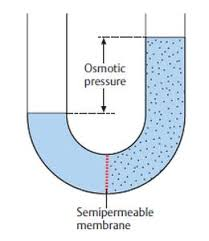The image above represents the osmotic pressure.

To compute for the osmotic pressure, five parameters are needed and these parameters are Ideal Gas Constant (R)Temperature in Kelvin (T), Number of Moles (n), Volume (V) and Van’t Hoff’s Factor (i).

The formula for calculating osmotic pressure:

π = i nRTV

Where;
π = osmotic pressure
n = number of moles
R = ideal gas constant
T = temperature in Kelvin
i = Van’t Hoff’s Factor
V = Volume

Let’s solve an example;
Find the osmotic pressure when the ideal gas constant is 0.08206 with a temperature in kelvin of 120, number of moles is 32, a volume of 48 and a van’t hoff’s factor of 24.

This implies that;
n = number of moles = 32
R = ideal gas constant = 0.08206
T = temperature in Kelvin = 120
i = Van’t Hoff’s Factor = 24
V = Volume = 48

π = i nRTV
π = 24 32 x 0.08206 x 12048
π = (24) (315.110)(48)
π = (24)(6.5647)
π = 157.5

Therefore, the osmotic pressure is 157.5 atm.

Calculating the Van’t Hoff’s Factor using the Osmotic Pressure, Number of Moles, Temperature in Kelvin, Ideal Gas Constant and Volume.

i = / nRT

Where;
i = Van’t Hoff’s Factor
π = osmotic pressure
V = Volume
n = number of moles
R = ideal gas constant
T = temperature in Kelvin

Let’s solve an example;
Find the Van’t Hoff’s Factor when the osmotic pressure is 220, volume of 50, temperature in kelvin of 180 and number of moles of 60. (R = 0.08206)

This implies that;
π = osmotic pressure = 220
V = Volume = 50
n = number of moles = 60
R = ideal gas constant = 0.08206
T = temperature in Kelvin = 180

i = / nRT
i = 50 x 220 / 60 x 0.08206 x 180
i = 11000 / 866.808
i = 12.69

Therefore, the Van’t Hoff’s Factor is 12.69.

Calculating the Volume using the Osmotic Pressure, Number of Moles, Temperature in Kelvin, Ideal Gas Constant and Van’t Hoff’s Factor.

V = i (nRT) / π

Where;
V = Volume
i = Van’t Hoff’s Factor
π = osmotic pressure
n = number of moles
R = ideal gas constant
T = temperature in Kelvin

Let’s solve an example;
Find the volume when the osmotic pressure is 280, Van’t Hoff’s Factor of 40, temperature in kelvin of 90 and number of moles of 70. (R = 0.08206)

This implies that;
i = Van’t Hoff’s Factor = 40
π = osmotic pressure = 280
n = number of moles = 70
R = ideal gas constant = 0.08206
T = temperature in Kelvin = 90

V = i (nRT) / π
V = 40 (70 x 0.08206 x 90) / 280
V = 40 (516.978) / 280
V = 20679.12 / 280
V = 73.854

Therefore, the volume is 73.854.

## How to Calculate and Solve for Van’t Hoff’s Factor, Cryoscopic Constant, Molality and Freezing Point Depression | Nickzom Calculator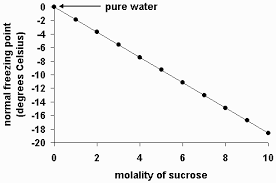The image above represents the freezing point depression.

To compute for the freezing point depression, three essential parameters are needed and these parameters are Van’t Hoff’s Factor (i), cryoscopic constant (Kf) and molality.

The formula for calculating freezing point depression:

δTf = iKf x Molality

Where;
δTf = Freezing point depression
i = Van’t Hoff’s Factor
Kf = cryoscopic constant
Molality

Let’s solve an example;
Find the freezing point depression when the van’t hoff’s factor is 12, cryoscopic constant is 21 with a molality of 16.

δTf = iKf x Molality
δTf = (12 x 21) x 16
δTf = 252 x 16
δTf = 4032

Therefore, the freezing point depression is 4032 °C m-1.

## How to Calculate and Solve for Mass, Volume and Density | The Calculator Encyclopedia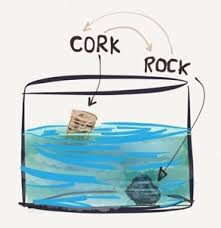The image above represents density.

To compute for the density, two essential parameters are needed and these parameters are mass (m) and volume (v).

The formula for calculating density:

Density = mass / volume

Let’s solve an example;
Given that the volume is 20 m³ with a mass of 240 kg. Find the density?

This implies that;
Volume = 20
Mass = 240

Density = mass / volume
Density = 240 / 20
Density = 12

Therefore, the density is 12 Kg/m³.

Calculating the Mass when the Density and Volume is Given.

Mass = Volume x Density

Let’s solve an example;
With a density of 90 kg/m³ and a volume of 15 m³, Find the mass?

This implies that;
Density = 90
Volume = 15

Mass = Volume x Density
Mass = 15 x 90
Mass = 1350

Therefore, the mass is 1350 kg.

## How to Apply Sine Rule, Cosine Rule and Tangent Rule in Trigonometry

### Sine Rule

In trigonometry, the sine law, law of sines, sine rule, or sine formula is an rational equation that relates to the lengths of the sides of a triangle (any shape or kind) to the sines of its angles.

According to the sine rule,

a / sin(A) = b / sin(B) = c / sin(C)

where a, b, and c are the lengths of the sides of a triangle, and A, B, and C are the opposite angles.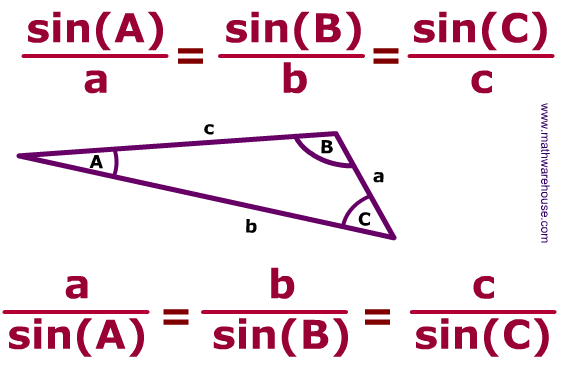Application of Sine Rule

• Sine rule can be used to find the unknown side or length of a triangle.
• Sine rule can also be used to find the unknown angle of a triangle.

To find the unknown side or length of a triangle, three essential parameters must be provided and these parameters are:

• The angle opposite to the unknown side or length of the triangle.
• The length of a side of the triangle (b).
• The angle opposite to the length of side (b) of the triangle.

Let’s take for example we have a triangle and we want to determine the unknown side of this triangle, when the opposite angle is given, another length of the side of the triangle and its opposite angle is also given. Like the image below.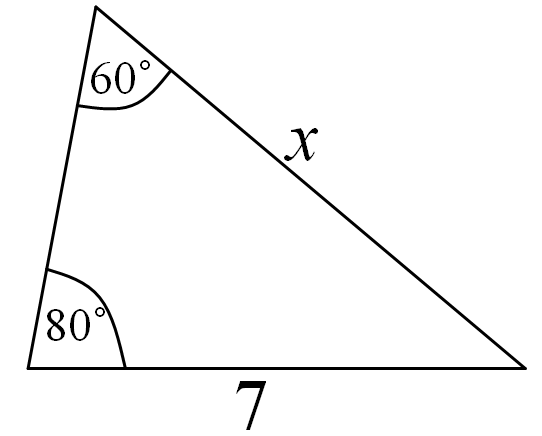From the image above, one can see that;
The angle opposite to the unknown length of the side of the triangle is 80°. (A)
The length of the side of the triangle given is 7. (b)
The angle opposite to the length of the side of the triangle given is 60° (B)
The unknown length of the side of the triangle is x. (a)

Applying the sine rule to find the value of x:

a / sin(A) = b / sin(B)

x / sin(80°) = 7 / sin(60°)
x / 0.9848 = 7 / 0.8660

Applying cross multiplication

x (0.8660) = 7 (0.9848)
x (0.8660) = 6.8936

Dividing both sides by 0.8660

x = 6.8936 / 0.8660
x = 7.96

Therefore, the unknown length of the side of the triangle, x is 7.96.